# 6.7 SPM Practice (Long Questions)

Question 10 (12 marks):
Diagram shows a histogram which represents the mass, in kg, for a group of 100 students.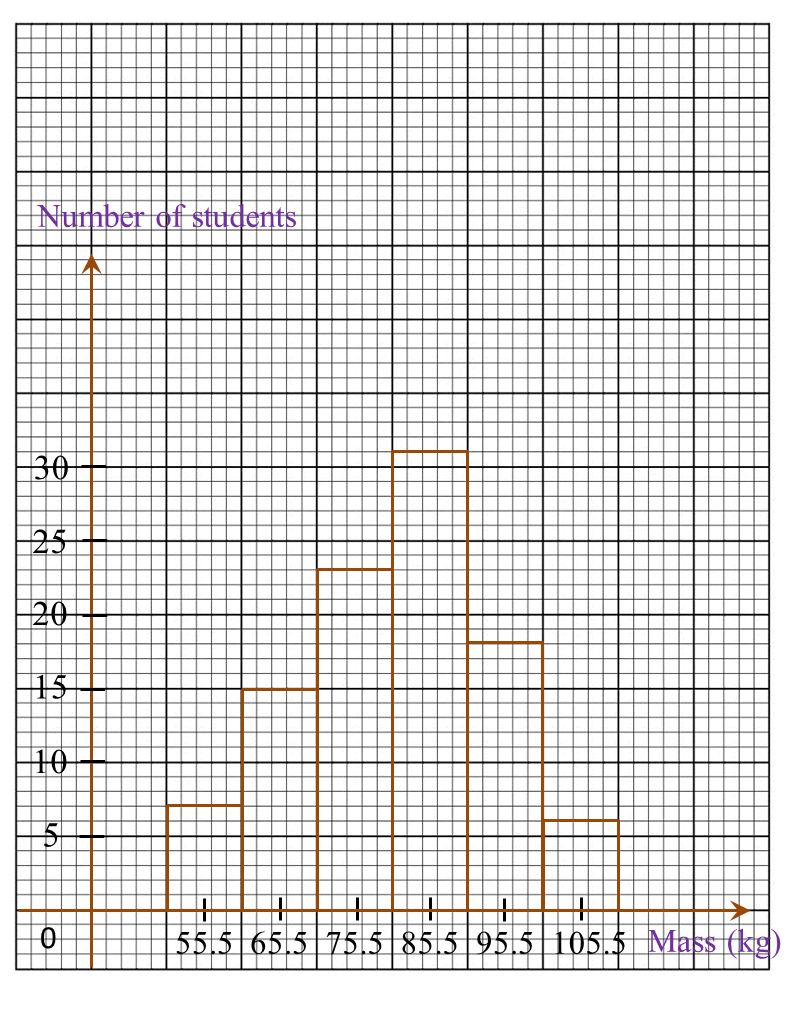Diagram

(a)
Based on the Diagram, complete the Table in the answer space.

(b)
Calculate the estimated mean mass of a student.

(c)
For this part of the question, use graph paper. You may use a flexible curve ruler.
Using a scale of 2 cm to 10 kg on the horizontal axis and 2 cm to 10 students on the vertical axis, draw an ogive for the data.

(d)
Based on the ogive drawn in 10(c), state the third quartile.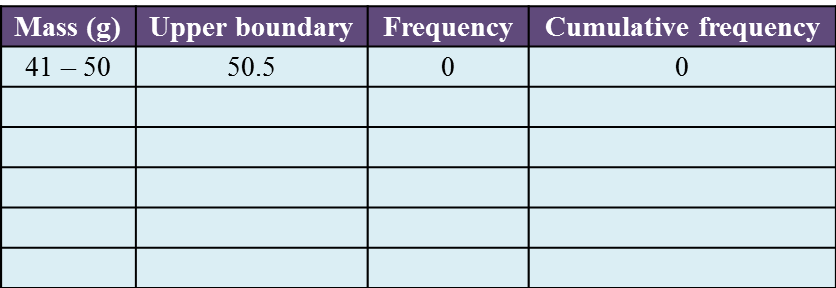Solution:
(a)(b)(c)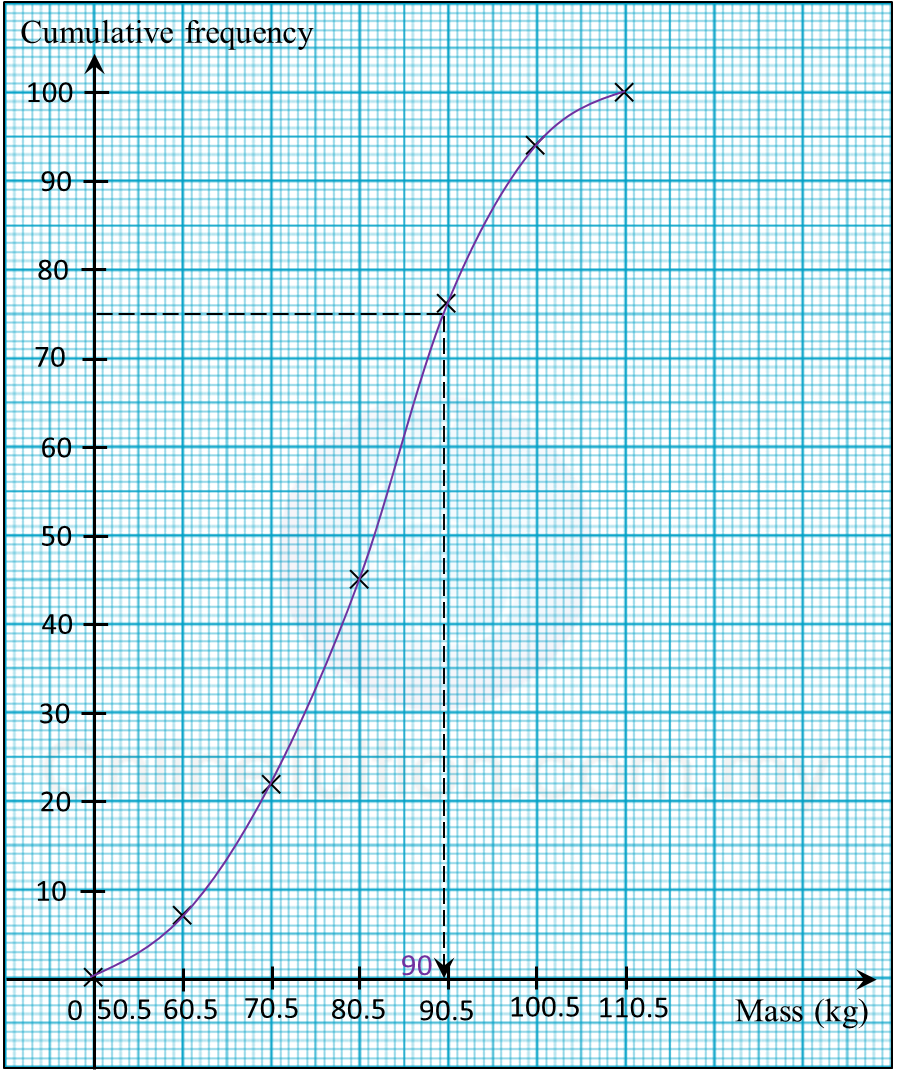(d)
Third quartile
= 75th student
= 90.0 kg

# 6.7 SPM Practice (Long Questions)

Question 9 (12 marks):
The data in the Diagram shows the mass, in g, of 30 strawberries plucked by a tourist from a farm.Diagram

(a)
Based on the Diagram, complete Table 3 in the answer space.

(b)
Based on Table, calculate the estimated mean mass of a strawberry.

(c)
For this part of the question, use graph paper.
By using the scale of 2 cm to 10 g on the horizontal axis and 2 cm to 1 strawberry on the vertical axis, draw a histogram for the data.

(d)
Based on the histogram drawn in 14(c), state the number of strawberries with the mass of more than 50 g.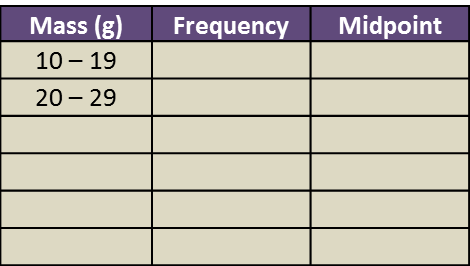Solution:
(a)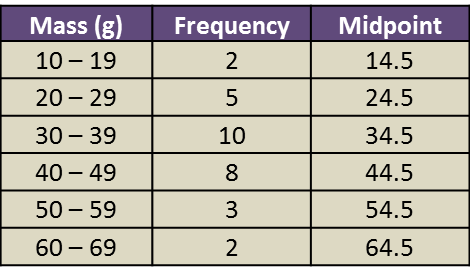(b)

(c)(d)
Number of strawberries with the mass more than 50 g
= 3 + 2
= 5

# 6.7 SPM Practice (Long Questions)

Question 8 (12 marks):
Diagram shows the marks obtained by a group of 36 students in a Mathematics test.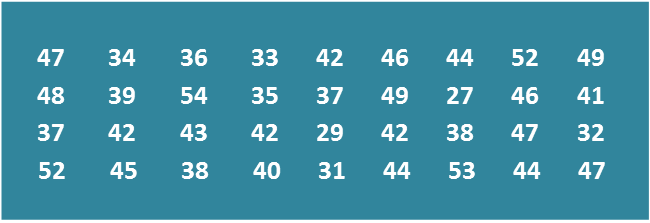Diagram

(a)
Based on the data in Diagram, complete Table in the answer space.

(b)
Based on the Table, calculate the estimated mean mark of a student.

(c)
For this part of the question, use graph paper.
By using the scale of 2 cm to 5 marks on the horizontal axis and 2 cm to 1 student on the vertical axis, draw a frequency polygon for the data.

(d)
Based on the frequency polygon in 8(c), state the number of students who obtained more than 40 marks.Table

Solution:
(a)(b)

(c)(d)
Number of students who obtained more than 40 marks
= 10 + 8 + 4
= 22 students

# 6.7 SPM Practice (Long Questions)

Question 7:
Diagram below shows the marks obtained by a group of 30 students in a history test.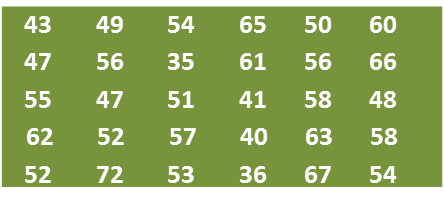(a) Using data in diagram above and a class interval of 5 marks, complete the table in the answer space.

(b) Based on the completed table in part (a)
(i) find the modal class,
(ii) calculate the mean mark obtained by the students.

(c) By using a scale of 2 cm to 10 marks on the horizontal axis and 2 cm to 1 student on the vertical axis, draw a frequency polygon for the data.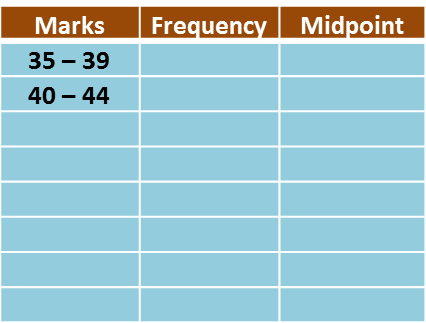Solution:
(a)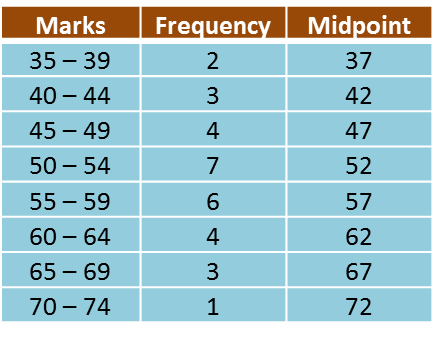(b)(i)
Modal class = 50 – 54

(b)(ii)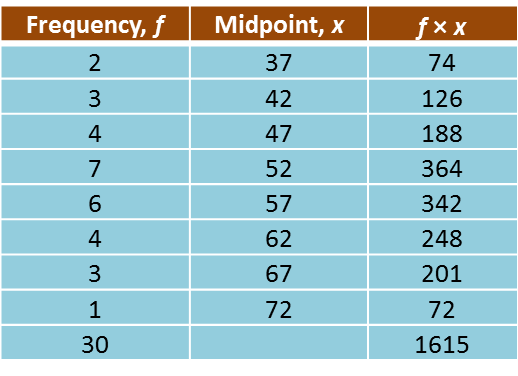(c)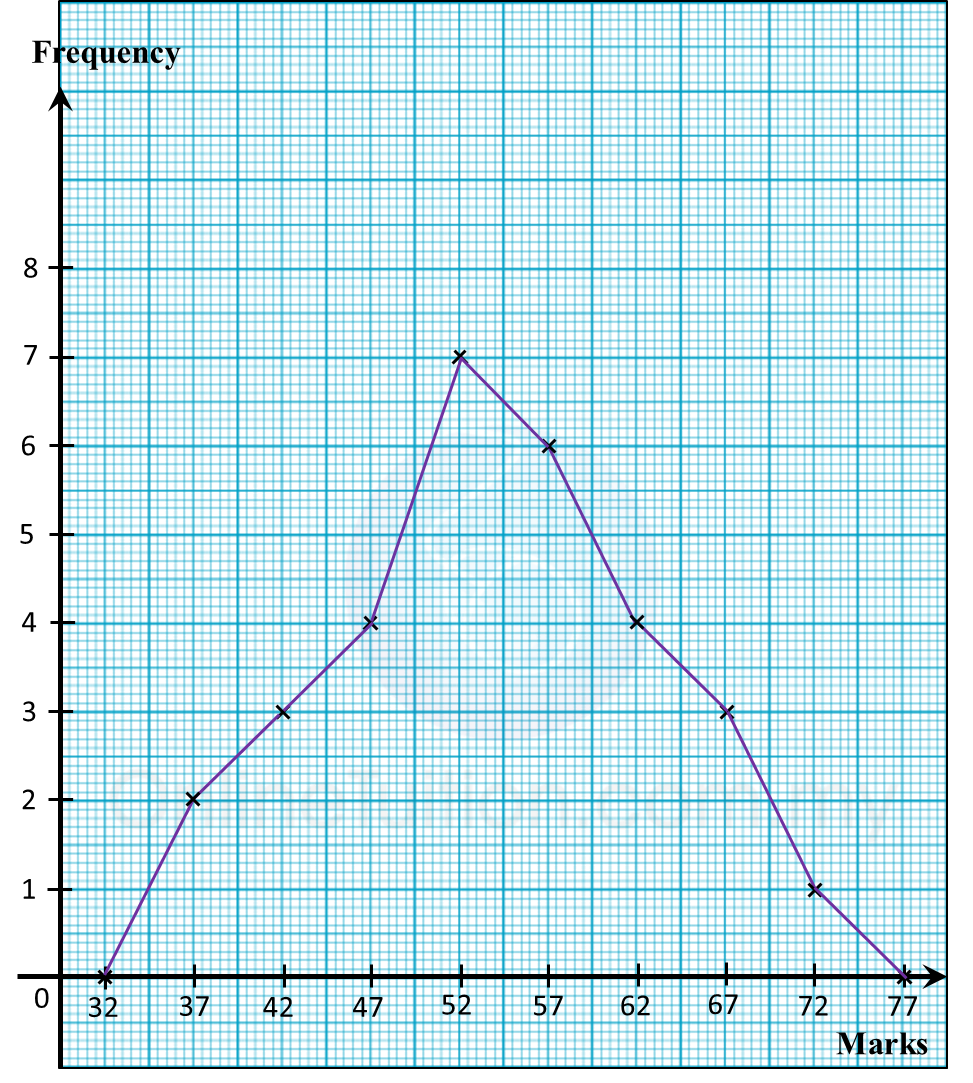# 6.7 SPM Practice (Long Questions)

Question 6:
The table below shows the frequency distribution of the age of 100 scouts in a camping spot.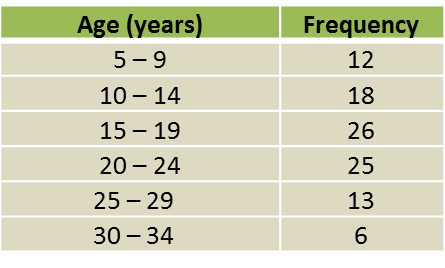(a) Based on table above, complete table in the answer space.

(b)
Calculate the estimated age, in years, of a scout in the camping spot.

(c)
For this part of the question, use graph paper. You may use a flexible curve rule.
By using a scale of 2 cm to 5 years on the horizontal axis and 2 cm to 10 scouts on the vertical axis, draw an ogive for the data.

(d)
Any scout which is older than 18 years old is considered senior scout.
Based on the ogive drawn in part (c), find the percentage of senior scout in the camping spot.Solution:
(a)(b)

(c)(d)

# 6.7 SPM Practice (Long Questions)

Question 5:
The table below shows the frequency distribution of the time spent by 50 swimmers in the pool in a swimming practice.

 Time (seconds) Frequency 35 – 39 5 40 – 44 8 45 – 49 9 50 – 54 15 55 – 59 11 60 – 64 2
(a) State the modal class.

(b) Calculate the estimated mean of the time spent of a swimmer.

(c) Based on table above, complete table below in the answer space by writing down the values of the upper boundary and the cumulative  frequency.

 Upper Boundary Cumulative Frequency 34.5 0 39.5 64.5 50
(d) For this part of the question, use graph paper. You may use a flexible curve rule.
By using a scale of 2 cm to 5 seconds on the horizontal axis and 2 cm to 5 swimmers on the vertical axis, draw an ogive for the data.

Solution:
(a) Modal class = time 50 – 54 seconds (highest frequency).

(b)

$\begin{array}{l}\text{Estimated mean}\\ \text{=}\frac{37×5+42×8+47×9+52×15+57×11+62×2}{50}\\ \text{=}\frac{2475}{50}=49.5\end{array}$

(c)

 Upper Boundary Cumulative Frequency 34.5 0 39.5 5 44.5 13 49.5 22 54.5 37 59.5 48 64.5 50

(d)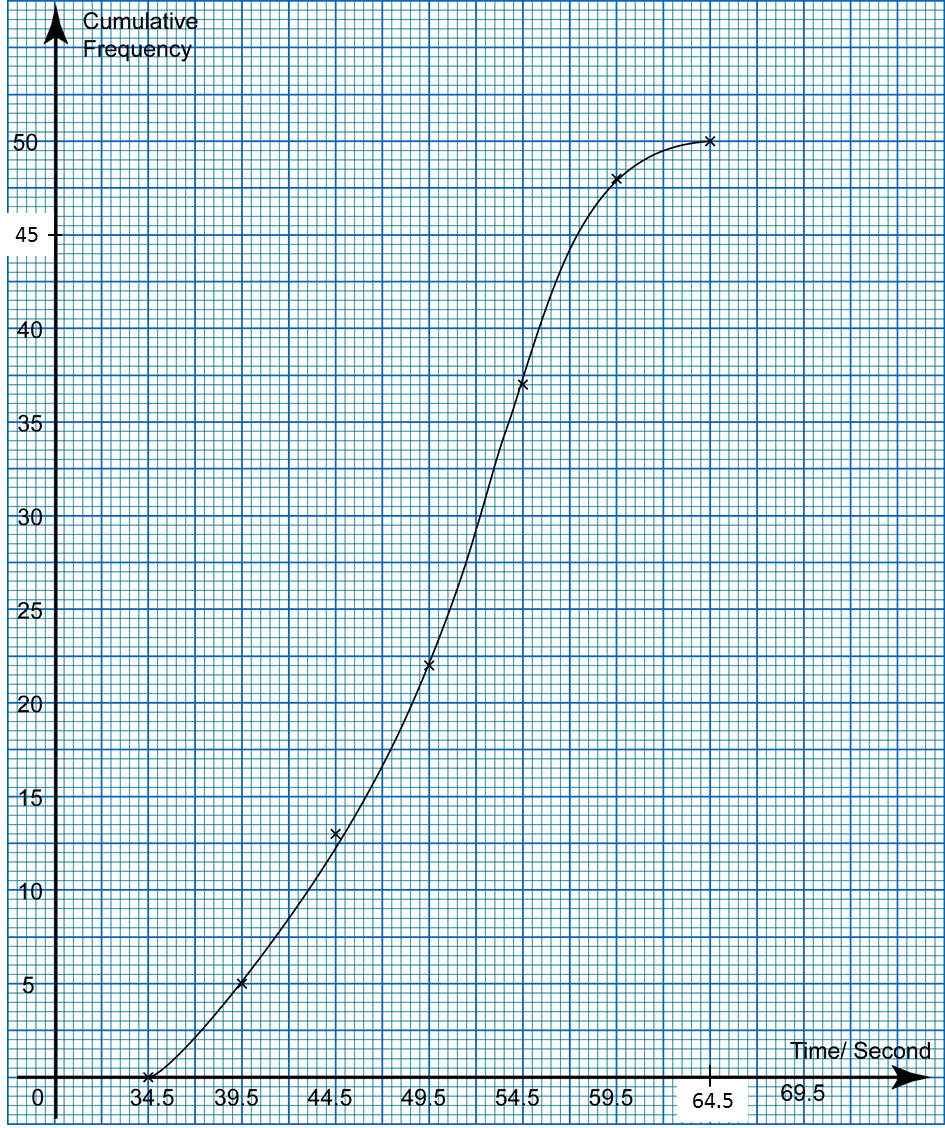# 6.7 SPM Practice (Long Questions)

Question 4:
Diagram below shows the marks obtained by a group of 30 students in a Science test.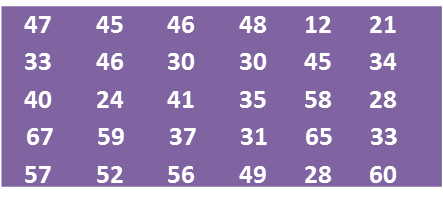(a) Based on the data in diagram above, complete the table in the answer space.

(b) Based on the completed table in part (a), calculate the estimated mean mark of a student.

For this part of the question, use graph paper.
(c) By using a scale of 2 cm to 10 marks on the horizontal axis and 2 cm to 1 student on the vertical axis, draw a frequency polygon for the data.

(d) The passing mark for the test is 44. Using the frequency polygon drawn in part (c), find the number of students who passed the test.Solution:
(a)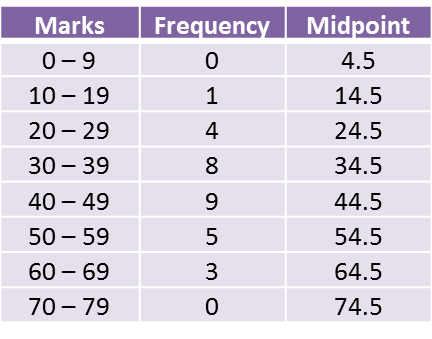(b)(c)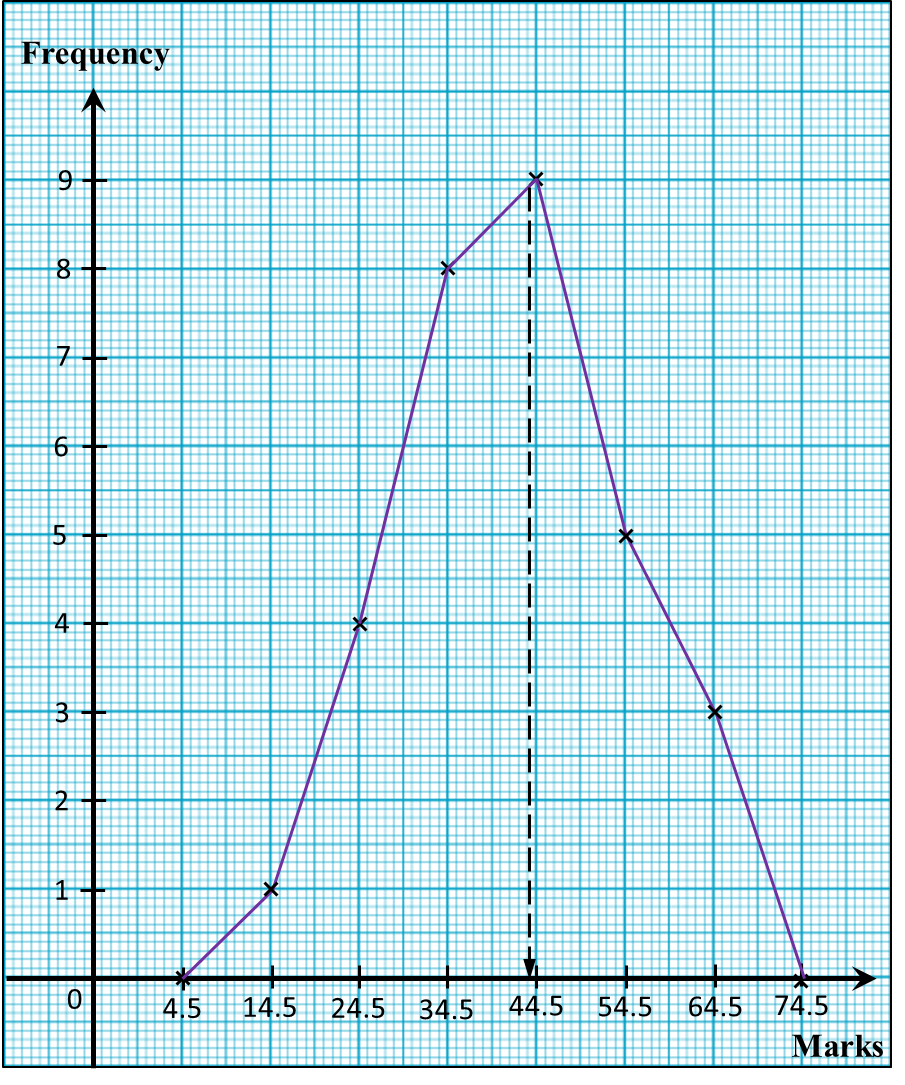(d)
Number of students who passed the test
= 9 + 5 + 3
= 17

# 6.6 Measures of Dispersion (Part 2)

(B) Medians and Quartiles
1. The first quartile (Q1)is a number such that 1 4 of the total number of data that has a value less than the number.

2.
The median is the second quartile which is the value that lies at the centre of the data.

3. The third quartile (Q3) is a number such that 3 4of the total number of data that has a value less than the number.

4. The interquartile range is the difference between the third quartile and the first quartile.

 Interquartile range = third quartile – first quartile

Example 2: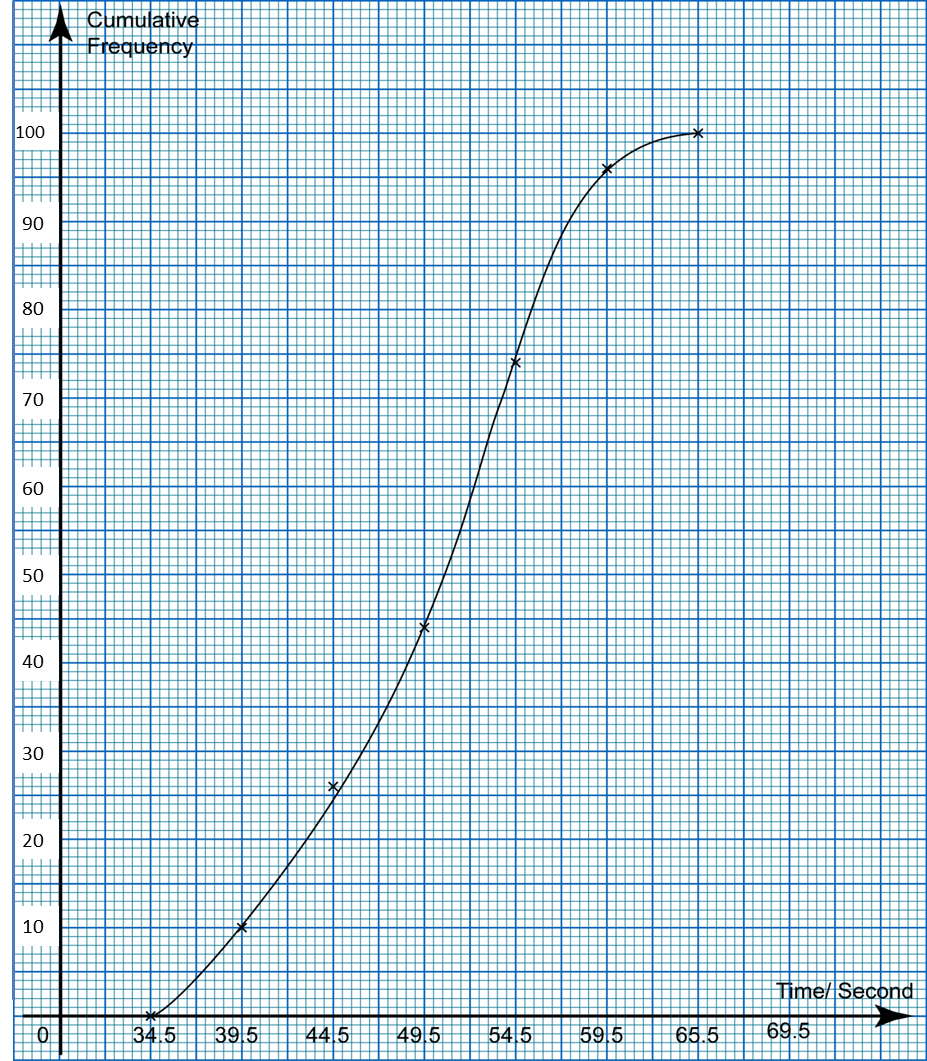The ogive in the diagram shows the distribution of time (to the nearest second) taken by 100 students in a swimming competition. From the ogive, determine
(a) the median,
(b) the first quartile,
(c) the third quartile
(d) the interquartile range of the time taken.

Solution:$\begin{array}{l}\text{(a)}\frac{1}{2}\text{of 100 students}=\frac{1}{2}×100=50\\ \text{From the ogive, median,}M=50.5\text{second}\\ \\ \text{(b)}\frac{1}{4}\text{of 100 students}=\frac{1}{4}×100=25\\ \text{From the ogive, first quartile,}{Q}_{1}\text{=}44.5\text{second}\\ \\ \text{(c)}\frac{3}{4}\text{of 100 students}=\frac{3}{4}×100=75\\ \text{From the ogive, third quartile,}{Q}_{3}\text{= 5}4.5\text{second}\end{array}$

(d)
Interquartile range
= Third quartile – First quartile
= 54.5 – 44.5
= 10.0 second

# 6.2 Mode and Mean of Grouped Data (Examples)

Example:
The following frequency table shows the number of magazines sold at a bookshop for 30 days in April 2013.

 Number of magazines Frequency 220 – 229 3 230 – 239 5 240 – 249 11 250 – 259 6 260 – 269 5
Based on the data given,
(a) calculate the size of class,
(b) state the modal class,
(c) calculate the mean number of magazine sold per day.

Solution:
(a) Size of the class
= upper boundary – lower boundary
= 229.5 – 219.5
= 10

(b) Modal class = 240 – 249 (Highest frequency)

(c)
 Number of magazines Frequency (f) Class midpoint (x) 220 – 229 3 224.5 230 – 239 5 234.5 240 – 249 11 244.5 250 – 259 6 254.5 260 – 269 5 264.5

# 6.5 Cumulative Frequency

6.5 Cumulative Frequency
Cumulative Frequency of a data or a class interval in a frequency table is obtained by determining the sum of its frequency with the total frequencies of all its previous data or class interval.

(A) Ogive
Ogive is a cumulative frequency graph which is obtained by plotting the cumulative frequency against the upper boundaries of each class.

Example:
The data below shows the number of books read by a group of 60 students in a year.

 Books Frequency 6-10 3 11-15 7 16-20 11 21-25 16 26-30 11 31-35 8 36-40 4
(a) Construct a cumulative frequency table for the given data.
(b) By using the scales of 2 cm to 5 books on the horizontal axis and 2 cm to 10 students on the vertical axis, draw an ogive for the data.

Solution:
(a)
• Add a class with frequency 0 before the first class.
• Find the upper boundary of each class interval.

 Books Frequency Cumulative Frequency Upper Boundary 1-5 0 0 5.5 6-10 3 0 + 3 = 3 10.5 11-15 7 3 + 7 = 10 15.5 16-20 11 10 + 11 = 21 20.5 21-25 16 21 + 16 = 37 25.5 26-30 11 37 + 11 = 48 30.5 31-35 8 48 + 8 = 56 35.5 36-40 4 56 + 4 = 60 40.5
(b)